# 查找算法 -- 简介

2.动态查找表(Dynamic Search Table)：在查找中同时进行插入或删除等操作： ①查找时插入数据 ②查找时删除数据

# 顺序查找

# 最基础的遍历无序列表的查找算法# 时间复杂度O(n)def sequential_search(lis, key):  length = len(lis)  for i in range(length):    if lis[i] == key:      return i    else:      return Falseif __name__ == '__main__':  LIST = [1, 5, 8, 123, 22, 54, 7, 99, 300, 222]  result = sequential_search(LIST, 123)  print(result)

# 二分查找

# 针对有序查找表的二分查找算法 def binary_search(lis, key):  low = 0  high = len(lis) - 1  time = 0  while low < high:    time += 1    mid = int((low + high) / 2)    if key < lis[mid]:      high = mid - 1    elif key > lis[mid]:      low = mid + 1    else:      # 打印折半的次数      print("times: %s" % time)      return mid  print("times: %s" % time)  return False if __name__ == '__main__':  LIST = [1, 5, 7, 8, 22, 54, 99, 123, 200, 222, 444]  result = binary_search(LIST, 99)  print(result)

# 插值查找

# 插值查找算法def binary_search(lis, key):  low = 0  high = len(lis) - 1  time = 0  while low < high:    time += 1    # 计算mid值是插值算法的核心代码    mid = low + int((high - low) * (key - lis[low])/(lis[high] - lis[low]))    print("mid=%s, low=%s, high=%s" % (mid, low, high))    if key < lis[mid]:      high = mid - 1    elif key > lis[mid]:      low = mid + 1    else:      # 打印查找的次数      print("times: %s" % time)      return mid  print("times: %s" % time)  return Falseif __name__ == '__main__':  LIST = [1, 5, 7, 8, 22, 54, 99, 123, 200, 222, 444]  result = binary_search(LIST, 444)  print(result)

# 斐波那契查找

# 斐波那契查找算法# 时间复杂度O(log(n)) def fibonacci_search(lis, key):  # 需要一个现成的斐波那契列表。其最大元素的值必须超过查找表中元素个数的数值。  F = [1, 1, 2, 3, 5, 8, 13, 21, 34, 55, 89, 144,     233, 377, 610, 987, 1597, 2584, 4181, 6765,     10946, 17711, 28657, 46368]  low = 0  high = len(lis) - 1     # 为了使得查找表满足斐波那契特性，在表的最后添加几个同样的值  # 这个值是原查找表的最后那个元素的值  # 添加的个数由F[k]-1-high决定  k = 0  while high > F[k]-1:    k += 1  print(k)  i = high  while F[k]-1 > i:    lis.append(lis[high])    i += 1  print(lis)     # 算法主逻辑。time用于展示循环的次数。  time = 0  while low <= high:    time += 1    # 为了防止F列表下标溢出，设置if和else    if k < 2:      mid = low    else:      mid = low + F[k-1]-1         print("low=%s, mid=%s, high=%s" % (low, mid, high))    if key < lis[mid]:      high = mid - 1      k -= 1    elif key > lis[mid]:      low = mid + 1      k -= 2    else:      if mid <= high:        # 打印查找的次数        print("times: %s" % time)        return mid      else:        print("times: %s" % time)        return high  print("times: %s" % time)  return False if __name__ == '__main__':  LIST = [1, 5, 7, 8, 22, 54, 99, 123, 200, 222, 444]  result = fibonacci_search(LIST, 444)  print(result)

# 树表查找

1、二叉树查找算法。 算法简介 二叉查找树是先对待查找的数据进行生成树，确保树的左分支的值小于右分支的值，然后在就行和每个节点的父节点比较大小，查找最适合的范围。 这个算法的查找效率很高，但是如果使用这种查找方法要首先创建树。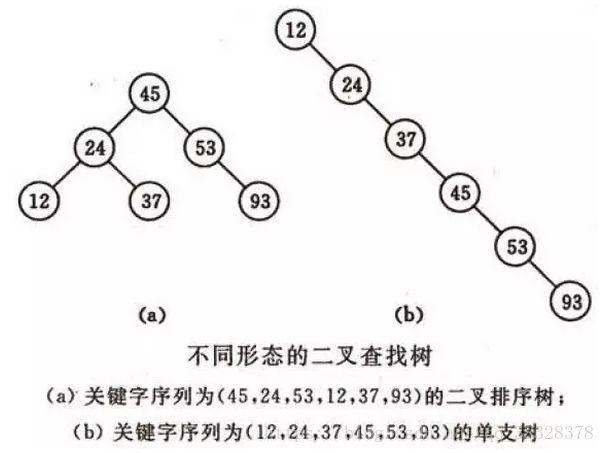# 二叉树查找 Python实现class BSTNode:    """    定义一个二叉树节点类。    以讨论算法为主，忽略了一些诸如对数据类型进行判断的问题。    """    def __init__(self, data, left=None, right=None):        """        初始化        :param data: 节点储存的数据        :param left: 节点左子树        :param right: 节点右子树        """        self.data = data        self.left = left        self.right = rightclass BinarySortTree:    """    基于BSTNode类的二叉查找树。维护一个根节点的指针。    """    def __init__(self):        self._root = None    def is_empty(self):        return self._root is None    def search(self, key):        """        关键码检索        :param key: 关键码        :return: 查询节点或None        """        bt = self._root        while bt:            entry = bt.data            if key < entry:                bt = bt.left            elif key > entry:                bt = bt.right            else:                return entry        return None    def insert(self, key):        """        插入操作        :param key:关键码         :return: 布尔值        """        bt = self._root        if not bt:            self._root = BSTNode(key)            return        while True:            entry = bt.data            if key < entry:                if bt.left is None:                    bt.left = BSTNode(key)                    return                bt = bt.left            elif key > entry:                if bt.right is None:                    bt.right = BSTNode(key)                    return                bt = bt.right            else:                bt.data = key                return    def delete(self, key):        """        二叉查找树最复杂的方法        :param key: 关键码        :return: 布尔值        """        p, q = None, self._root     # 维持p为q的父节点，用于后面的链接操作        if not q:            print("空树！")            return        while q and q.data != key:            p = q            if key < q.data:                q = q.left            else:                q = q.right            if not q:               # 当树中没有关键码key时，结束退出。                return        # 上面已将找到了要删除的节点，用q引用。而p则是q的父节点或者None(q为根节点时)。        if not q.left:            if p is None:                self._root = q.right            elif q is p.left:                p.left = q.right            else:                p.right = q.right            return        # 查找节点q的左子树的最右节点，将q的右子树链接为该节点的右子树        # 该方法可能会增大树的深度，效率并不算高。可以设计其它的方法。        r = q.left        while r.right:            r = r.right        r.right = q.right        if p is None:            self._root = q.left        elif p.left is q:            p.left = q.left        else:            p.right = q.left    def __iter__(self):        """        实现二叉树的中序遍历算法,        展示我们创建的二叉查找树.        直接使用python内置的列表作为一个栈。        :return: data        """        stack = []        node = self._root        while node or stack:            while node:                stack.append(node)                node = node.left            node = stack.pop()            yield node.data            node = node.rightif __name__ == '__main__':    lis = [62, 58, 88, 48, 73, 99, 35, 51, 93, 29, 37, 49, 56, 36, 50]    bs_tree = BinarySortTree()    for i in range(len(lis)):        bs_tree.insert(lis[i])    # bs_tree.insert(100)    bs_tree.delete(58)    for i in bs_tree:        print(i, end=" ")    # print("", bs_tree.search(4))

2、平衡查找树之2-3查找树(2-3 Tree) 2-3查找树定义 和二叉树不一样，2-3树运行每个节点保存1个或者两个的值。对于普通的2节点(2-node)，他保存1个key和左右两个自己点。对应3节点(3-node)，保存两个Key，2-3查找树的定义如下： 　　 1)要么为空，要么： 　　 2)对于2节点，该节点保存一个key及对应value，以及两个指向左右节点的节点，左节点也是一个2-3节点，所有的值都比key要小，右节点也是一个2-3节点，所有的值比key要大。 　　 3)对于3节点，该节点保存两个key及对应value，以及三个指向左中右的节点。左节点也是一个2-3节点，所有的值均比两个key中的最小的key还要小；中间节点也是一个2-3节点，中间节点的key值在两个跟节点key值之间；右节点也是一个2-3节点，节点的所有key值比两个key中的最大的key还要大。

2-3查找树的性质 　　 1)如果中序遍历2-3查找树，就可以得到排好序的序列； 　　 2)在一个完全平衡的2-3查找树中，根节点到每一个为空节点的距离都相同。(这也是平衡树中“平衡”一词的概念，根节点到叶节点的最长距离对应于查找算法的最坏情况，而平衡树中根节点到叶节点的距离都一样，最坏情况也具有对数复杂度。) 复杂度分析： 　　 2-3树的查找效率与树的高度是息息相关的。 距离来说，对于1百万个节点的2-3树，树的高度为12-20之间，对于10亿个节点的2-3树，树的高度为18-30之间。 　　 对于插入来说，只需要常数次操作即可完成，因为他只需要修改与该节点关联的节点即可，不需要检查其他节点，所以效率和查找类似。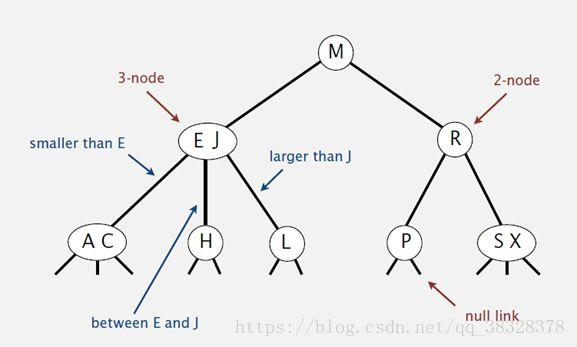class Node(object):    def __init__(self,key):        self.key1=key        self.key2=None        self.left=None        self.middle=None        self.right=None    def isLeaf(self):        return self.left is None and self.middle is None and self.right is None    def isFull(self):        return self.key2 is not None    def hasKey(self,key):        if (self.key1==key) or (self.key2 is not None and self.key2==key):            return True        else:            return False    def getChild(self,key):        if key

3、平衡查找树之红黑树(Red-Black Tree) 红黑树的定义 　　 红黑树是一种具有红色和黑色链接的平衡查找树，同时满足： 　　 ① 红色节点向左倾斜 ； 　　 ②一个节点不可能有两个红色链接； 　　 ③整个树完全黑色平衡，即从根节点到所以叶子结点的路径上，黑色链接的个数都相同。 红黑树的性质 整个树完全黑色平衡，即从根节点到所以叶子结点的路径上，黑色链接的个数都相同(2-3树的第2)性质，从根节点到叶子节点的距离都相等)。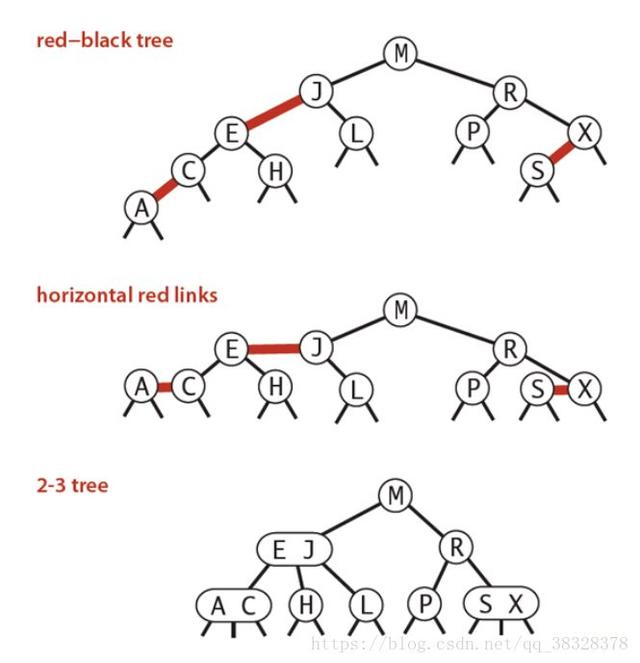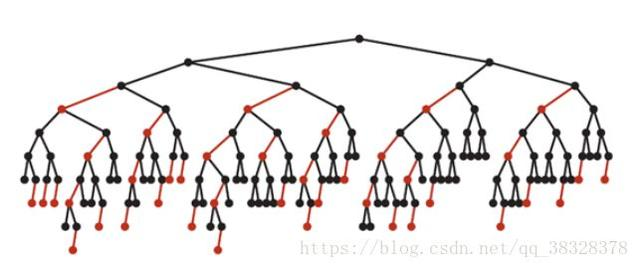#红黑树from random import randintRED = 'red'BLACK = 'black'class RBT:    def __init__(self):       # self.items = []        self.root = None        self.zlist = []    def LEFT_ROTATE(self, x):        # x是一个RBTnode        y = x.right        if y is None:            # 右节点为空，不旋转            return        else:            beta = y.left            x.right = beta            if beta is not None:                beta.parent = x            p = x.parent            y.parent = p            if p is None:                # x原来是root                self.root = y            elif x == p.left:                p.left = y            else:                p.right = y            y.left = x            x.parent = y    def RIGHT_ROTATE(self, y):        # y是一个节点        x = y.left        if x is None:            # 右节点为空，不旋转            return        else:            beta = x.right            y.left = beta            if beta is not None:                beta.parent = y            p = y.parent            x.parent = p            if p is None:                # y原来是root                self.root = x            elif y == p.left:                p.left = x            else:                p.right = x            x.right = y            y.parent = x    def INSERT(self, val):        z = RBTnode(val)        y = None        x = self.root        while x is not None:            y = x            if z.val < x.val:                x = x.left            else:                x = x.right        z.PAINT(RED)        z.parent = y        if y is None:            # 插入z之前为空的RBT            self.root = z            self.INSERT_FIXUP(z)            return        if z.val < y.val:            y.left = z        else:            y.right = z        if y.color == RED:            # z的父节点y为红色，需要fixup。            # 如果z的父节点y为黑色，则不用调整            self.INSERT_FIXUP(z)        else:            return    def INSERT_FIXUP(self, z):        # case 1:z为root节点        if z.parent is None:            z.PAINT(BLACK)            self.root = z            return        # case 2:z的父节点为黑色        if z.parent.color == BLACK:            # 包括了z处于第二层的情况            # 这里感觉不必要啊。。似乎z.parent为黑色则不会进入fixup阶段            return        # 下面的几种情况，都是z.parent.color == RED:        # 节点y为z的uncle        p = z.parent        g = p.parent  # g为x的grandpa        if g is None:            return            #   return 这里不能return的。。。        if g.right == p:            y = g.left        else:            y = g.right        # case 3-0:z没有叔叔。即：y为NIL节点        # 注意，此时z的父节点一定是RED        if y == None:            if z == p.right and p == p.parent.left:                # 3-0-0:z为右儿子,且p为左儿子，则把p左旋                # 转化为3-0-1或3-0-2的情况                self.LEFT_ROTATE(p)                p, z = z, p                g = p.parent            elif z == p.left and p == p.parent.right:                self.RIGHT_ROTATE(p)                p, z = z, p            g.PAINT(RED)            p.PAINT(BLACK)            if p == g.left:                # 3-0-1:p为g的左儿子                self.RIGHT_ROTATE(g)            else:                # 3-0-2:p为g的右儿子                self.LEFT_ROTATE(g)            return        # case 3-1:z有黑叔        elif y.color == BLACK:            if p.right == z and p.parent.left == p:                # 3-1-0:z为右儿子,且p为左儿子,则左旋p                # 转化为3-1-1或3-1-2                self.LEFT_ROTATE(p)                p, z = z, p            elif p.left == z and p.parent.right == p:                self.RIGHT_ROTATE(p)                p, z = z, p            p = z.parent            g = p.parent            p.PAINT(BLACK)            g.PAINT(RED)            if p == g.left:                # 3-1-1:p为g的左儿子，则右旋g                self.RIGHT_ROTATE(g)            else:                # 3-1-2:p为g的右儿子，则左旋g                self.LEFT_ROTATE(g)            return        # case 3-2:z有红叔        # 则涂黑父和叔，涂红爷，g作为新的z，递归调用        else:            y.PAINT(BLACK)            p.PAINT(BLACK)            g.PAINT(RED)            new_z = g            self.INSERT_FIXUP(new_z)    def DELETE(self, val):        curNode = self.root        while curNode is not None:            if val < curNode.val:                curNode = curNode.left            elif val > curNode.val:                curNode = curNode.right            else:                # 找到了值为val的元素,正式开始删除                if curNode.left is None and curNode.right is None:                    # case1:curNode为叶子节点：直接删除即可                    if curNode == self.root:                        self.root = None                    else:                        p = curNode.parent                        if curNode == p.left:                            p.left = None                        else:                            p.right = None                elif curNode.left is not None and curNode.right is not None:                    sucNode = self.SUCCESOR(curNode)                    curNode.val, sucNode.val  = sucNode.val, curNode.val                    self.DELETE(sucNode.val)                else:                    p = curNode.parent                    if curNode.left is None:                        x = curNode.right                    else:                        x = curNode.left                    if curNode == p.left:                        p.left = x                    else:                        p.right = x                    x.parent = p                    if curNode.color == BLACK:                        self.DELETE_FIXUP(x)                curNode = None        return False    def DELETE_FIXUP(self, x):        p = x.parent        # w:x的兄弟结点        if x == p.left:            w = x.right        else:            w = x.left        # case1:x的兄弟w是红色的        if w.color == RED:            p.PAINT(RED)            w.PAINT(BLACK)            if w == p.right:                self.LEFT_ROTATE(p)            else:                self.RIGHT_ROTATE(p)        if w.color == BLACK:            # case2:x的兄弟w是黑色的，而且w的两个孩子都是黑色的            if w.left.color == BLACK and w.right.color == BLACK:                w.PAINT(RED)                if p.color == BLACK:                    return                else:                    p.color = BLACK                    self.DELETE_FIXUP(p)            # case3:x的兄弟w是黑色的，而且w的左儿子是红色的，右儿子是黑色的            if w.left.color == RED and w.color == BLACK:                w.left.PAINT(BLACK)                w.PAINT(RED)                self.RIGHT_ROTATE(w)            # case4:x的兄弟w是黑色的，而且w的右儿子是红            if w.right.color == RED:                p.PAINT(BLACK)                w.PAINT(RED)                if w == p.right:                    self.LEFT_ROTATE(p)                else:                    self.RIGHT_ROTATE(p)    def SHOW(self):        self.DISPLAY1(self.root)        return self.zlist    def DISPLAY1(self, node):        if node is None:            return        self.DISPLAY1(node.left)        self.zlist.append(node.val)        self.DISPLAY1(node.right)    def DISPLAY2(self, node):        if node is None:            return        self.DISPLAY2(node.left)        print(node.val)        self.DISPLAY2(node.right)    def DISPLAY3(self, node):        if node is None:            return        self.DISPLAY3(node.left)        self.DISPLAY3(node.right)        print(node.val)class RBTnode:    '''红黑树的节点类型'''    def __init__(self, val):        self.val = val        self.left = None        self.right = None        self.parent = None    def PAINT(self, color):        self.color = colordef zuoxuan(b, c):    a = b.parent    a.left = c    c.parent = a    b.parent = c    c.left = bif __name__ == '__main__':    rbt=RBT()    b = []    for i in range(100):        m = randint(0, 500)        rbt.INSERT(m)        b.append(m)    a = rbt.SHOW()    b.sort()    equal = True    for i in range(100):        if a[i] != b[i]:            equal = False            break    if not equal:        print('wrong')    else:        print('OK!')

4、B树和B+树(B Tree/B+ Tree) B树简介 B 树可以看作是对2-3查找树的一种扩展，即他允许每个节点有M-1个子节点。 ①根节点至少有两个子节点； ②每个节点有M-1个key，并且以升序排列； ③位于M-1和M key的子节点的值位于M-1 和M key对应的Value之间； ④非叶子结点的关键字个数=指向儿子的指针个数-1； ⑤非叶子结点的关键字：K, K, …, K[M-1]；且K[i] ； ⑥其它节点至少有M/2个子节点； ⑦所有叶子结点位于同一层； 如：(M=3)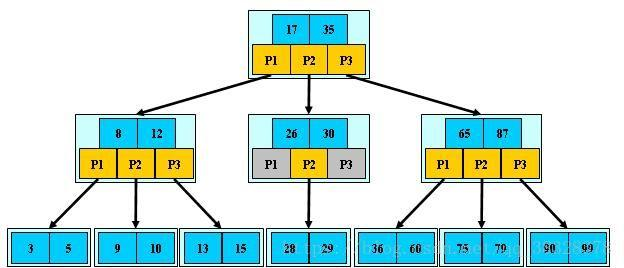B树算法思想 B-树的搜索，从根结点开始，对结点内的关键字(有序)序列进行二分查找，如果命中则结束，否则进入查询关键字所属范围的儿子结点；重复，直到所对应的儿子指针为空，或已经是叶子结点； B树的特性 1.关键字集合分布在整颗树中； 2.任何一个关键字出现且只出现在一个结点中； 3.搜索有可能在非叶子结点结束； 4.其搜索性能等价于在关键字全集内做一次二分查找； 5.自动层次控制； 由于限制了除根结点以外的非叶子结点，至少含有M/2个儿子，确保了结点的至少利用率，其最底搜索性能为O(LogN)

B+ 树简介

B+树是B-树的变体，也是一种多路搜索树： 1.其定义基本与B-树同，除了： 2.非叶子结点的子树指针与关键字个数相同； 3.非叶子结点的子树指针P[i]，指向关键字值属于[K[i], K[i+1])的子树 4.B-树是开区间； 5.为所有叶子结点增加一个链指针； 6.所有关键字都在叶子结点出现；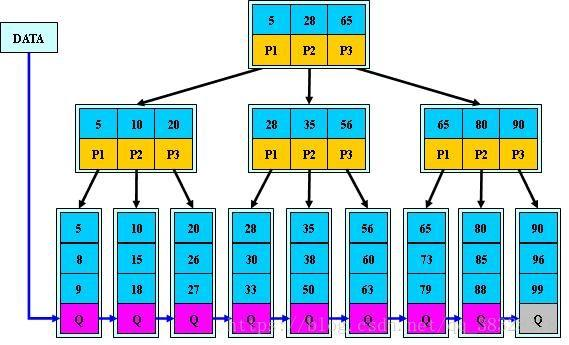B+树算法思想

B+的搜索与B-树也基本相同，区别是B+树只有达到叶子结点才命中(B-树可以在非叶子结点命中)，其性能也等价于在关键字全集做一次二分查找； B+树的特性 1.所有关键字都出现在叶子结点的链表中(稠密索引)，且链表中的关键字恰好是有序的； 2.不可能在非叶子结点命中； 3.非叶子结点相当于是叶子结点的索引(稀疏索引)，叶子结点相当于是存储(关键字)数据的数据层； 4.更适合文件索引系统； 算法实现

# -*- coding: UTF-8 -*-# B树查找class BTree:  #B树    def __init__(self,value):        self.left=None        self.data=value        self.right=None    def insertLeft(self,value):        self.left=BTree(value)        return self.left    def insertRight(self,value):        self.right=BTree(value)        return self.right    def show(self):        print(self.data)def inorder(node):  #中序遍历：先左子树，再根节点，再右子树    if node.data:        if node.left:            inorder(node.left)        node.show()        if node.right:            inorder(node.right)def rinorder(node):  #倒中序遍历    if node.data:        if node.right:            rinorder(node.right)        node.show()        if node.left:            rinorder(node.left)def insert(node,value):    if value > node.data:        if node.right:            insert(node.right,value)        else:            node.insertRight(value)    else:        if node.left:            insert(node.left,value)        else:            node.insertLeft(value)if __name__ == "__main__":    l=[88,11,2,33,22,4,55,33,221,34]    Root=BTree(l)    node=Root    for i in range(1,len(l)):        insert(Root,l[i])    print("中序遍历(从小到大排序 )")    inorder(Root)    print("倒中序遍历(从大到小排序)")    rinorder(Root)

5、树表查找总结 　　二叉查找树平均查找性能不错，为O(logn)，但是最坏情况会退化为O(n)。在二叉查找树的基础上进行优化，我们可以使用平衡查找树。平衡查找树中的2-3查找树，这种数据结构在插入之后能够进行自平衡操作，从而保证了树的高度在一定的范围内进而能够保证最坏情况下的时间复杂度。但是2-3查找树实现起来比较困难，红黑树是2-3树的一种简单高效的实现，他巧妙地使用颜色标记来替代2-3树中比较难处理的3-node节点问题。红黑树是一种比较高效的平衡查找树，应用非常广泛，很多编程语言的内部实现都或多或少的采用了红黑树。 　　除此之外，2-3查找树的另一个扩展——B/B+平衡树，在文件系统和数据库系统中有着广泛的应用。

# 分块查找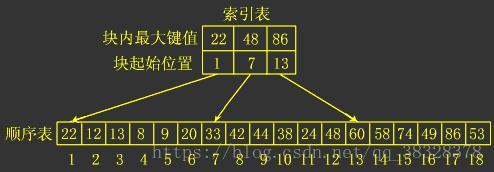# 哈希查找

1)用给定的哈希函数构造哈希表； 　　2)根据选择的冲突处理方法解决地址冲突； 　　　　 常见的解决冲突的方法：拉链法和线性探测法。 　　3)在哈希表的基础上执行哈希查找。 复杂度分析 　　单纯论查找复杂度：对于无冲突的Hash表而言，查找复杂度为O(1)(注意，在查找之前我们需要构建相应的Hash表)。

# 忽略了对数据类型，元素溢出等问题的判断。 class HashTable:  def __init__(self, size):    self.elem = [None for i in range(size)] # 使用list数据结构作为哈希表元素保存方法    self.count = size # 最大表长   def hash(self, key):    return key % self.count # 散列函数采用除留余数法   def insert_hash(self, key):    """插入关键字到哈希表内"""    address = self.hash(key) # 求散列地址    while self.elem[address]: # 当前位置已经有数据了，发生冲突。      address = (address+1) % self.count # 线性探测下一地址是否可用    self.elem[address] = key # 没有冲突则直接保存。   def search_hash(self, key):    """查找关键字，返回布尔值"""    star = address = self.hash(key)    while self.elem[address] != key:      address = (address + 1) % self.count      if not self.elem[address] or address == star: # 说明没找到或者循环到了开始的位置        return False    return True  if __name__ == '__main__':  list_a = [12, 67, 56, 16, 25, 37, 22, 29, 15, 47, 48, 34]  hash_table = HashTable(12)  for i in list_a:    hash_table.insert_hash(i)   for i in hash_table.elem:    if i:      print((i, hash_table.elem.index(i)), end=" ")  print("")   print(hash_table.search_hash(15))  print(hash_table.search_hash(33))1、点赞 + 评论 (勾选 “转发” )

2、关注小编私信。点击头像，关注。并私信回复关键词： 1024

01-01301-073
12-2347
12-2317
12-238
12-234
12-237
01-052
02-058
12-312
12-2318
12-275
01-1310
12-2810
12-2320
12-265
05-01
09-29
©️2020 CSDN 皮肤主题: 1024 设计师:白松林